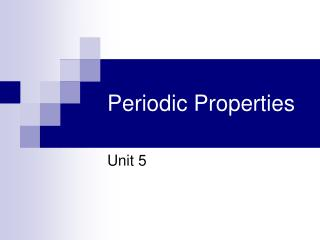Download PresentationPeriodic Properties

# Periodic Properties

Download Presentation## Periodic Properties

- - - - - - - - - - - - - - - - - - - - - - - - - - - E N D - - - - - - - - - - - - - - - - - - - - - - - - - - -
##### Presentation Transcript

1. Periodic Properties Unit 5

2. Pre-Lab Write-Up • The following are due before you can do the lab: • Title • Purpose (be specific) • Procedure (be specific) • Data Table(s) – Remember this is in the results section

3. Purpose • The purpose of this lab is to use the process of volume displacement to determine the density of three group IVA elements: Si, Sn, and Pb; and to use these values to estimate densities for C and Ge.

4. Materials • Goggles • Balance • 100/10 mL graduated cylinder • Silicon (5 g) • Tin (10 g) • Lead (25 g) • Weigh boats

5. Procedure 1.Using the balance measure out approximately 5g of silicon. Record the mass in your data table to the nearest .001 g. 2.Fill a 100 mL graduated cylinder about half way with water. Measure the volume to the nearest 0.1 mL and record in your data table. 3.Carefully add the silicon to the graduated cylinder. Make sure that the element is completely submerged. Measure this volume and record in your data table. 4.Repeat steps 1-3 using approximately 10g of Sn and 25g of Pb (It might be a good idea to replace the 100 mL cylinder with the 10 mL for these 2 elements).

6. Data Table Mass and Volumes of Group IVA Elements

7. Post-Lab Write-Up • The following will be completed once you have completed the lab: • Data Analysis/Calculations • Graph • Conclusion

8. Data Analysis/Calculations • Show calculation for the density of each element, include proper unit, and watch for sig. figs. • Density = mass (g) / ∆volume (mL) • Calculate the percent error of the density of each element. This will be done after the graph. Exp - Act Act % Error = x 100 Actual Density of C = 1.4 g/mL Actual Density of Ge = 5.3 g/mL

9. Graph • Construct a graph of density vs. atomic number (plot density on the y-axis and atomic number on the x-axis; both axes MUST start at zero.) • Draw a best fit line (or curve) through your data • Use the graph to interpolate the density for Ge and extrapolate the density for C • Include appropriate markings on the graph for the unknown densities • Follow all graphing techniques discussed in class

10. Conclusion • In your conclusion you should examine the results of your lab. • What values did you obtain for the densities of Ge and C? • How were you able to determine the densities of these two elements? Explain. • Were the results close to what they were supposed to be (examine your percent error)? • Why did you have error in your lab; what could you/would you do differently next time?